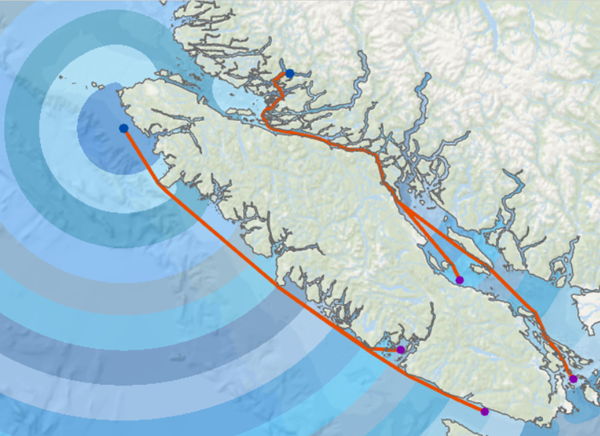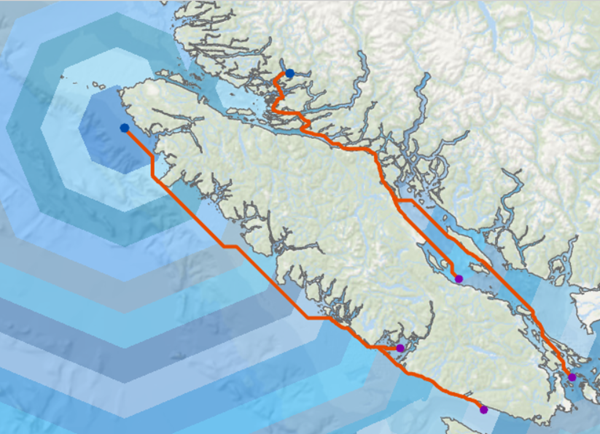# Migrating from legacy distance tools to distortion free distance tools

Significant improvements have been made to the Distance toolset and the distance raster functions in ArcGIS Pro 2.5. In addition to enhanced capabilities, the results of the operations are now calculated with greater precision and accuracy.

## Distortion free distance analysis

In ArcGIS Pro 2.5, a new algorithm for cost based distance mapping has been implemented. This algorithm removes the distortion in outputs caused by using a network model of cell connectivity. Removing this distortion provides the following benefits:

• Cost accumulation is measured the same way in all directions. An important special case is that cost distance with a constant cost surface now produces the same output as Euclidean distance mapping.
• Surface distance over a digital elevation model is accurately and precisely computed.
• Paths around barriers are followed accurately and precisely.

The difference between the new distortion free distance analysis and the legacy distance analysis can be demonstrated visually. The two images below compare the results from the different analytical methods.

The following image shows the output (blue bands) from the new Distance Accumulation tool with an input barrier, along with output (orange lines) from the new Optimal Path As Line tool:The image shows the distortion free distance accumulation raster with the optimal paths as lines.

The image below shows the output (blue bands) from the legacy Cost Distance tool using a constant input with barriers encoded as NoData, along with output (orange lines) from the legacy Cost Path As Polyline tool. As you can see, the smooth shapes generated by the new tools (above) are more representative of real-world conditions than those generated by the earlier tools (below).## Geodesic analysis

Geodesic cost distance analysis is available with the Distance Accumulation, Distance Allocation, and Optimal Region Connections tools.

## Intensity mapping

By using the new Out source location raster output from the Distance Accumulation and Distance Allocation tools, you can do intensity mapping of the boundary of sources, showing where most cost paths in the study area would end up, without plotting all cost paths. The Raster Cell Iterator python object, also new in ArcGIS Pro 2.5, makes it possible to perform this type of intensity calculation.

Using the new Optimal Path as Raster tool, you can count how many least cost paths pass through a cell en route from sources to destinations.

## Toolset organization and mapping

The original Distance tools that you are familiar with are still available and are located in the Legacy subtoolset. It is recommended that you use the new distortion free distance tools for your distance analysis workflows going forward.

The new tools are as follows:

• Distance Accumulation—Calculates accumulated distance for each cell to sources, allowing for straight-line distance, cost distance, and true surface distance, as well as vertical and horizontal cost factors.

• Distance Allocation—Calculates distance allocation for each cell to sources, allowing for straight-line distance, cost distance, and true surface distance, as well as vertical and horizontal cost factors.

• Optimal Path As Raster—Calculates the optimal path from a source to a destination as a raster.

• Optimal Path As Line—Calculates the optimal path from a source to a destination as a line.

• Optimal Region Connections—Connects regions in the most optimal way.

For new workflows, the back direction raster replaces the back link raster. The Optimal Path As Raster and the Optimal Path As Line tools do not accept a backlink raster as input (they will accept a flow direction raster). If you were using the backlink raster in a workflow that involves something other than the Cost Path or Cost Path As Polyline tools, contact the Spatial Analyst team.

If you used the Maximum Distance parameter in the legacy cost distance tools, use the Maximum Accumulation parameter in the Characteristics of the sources parameter group going forward.

## References

Goodchild, M. F. 1977. An Evaluation of Lattice Solutions to the Problem of Corridor Location. Environment and Planning A, Vol. 9, No. 7, 727-738.

Sethian, J. A. 1997. Tracking Interfaces with Level Sets: An “act of violence” helps solve evolving interface problems in geometry, fluid mechanics, robotic navigation and materials sciences. American Scientist, Vol. 85, No. 3, 254-263.

Sethian, J. A. 1999. Level set methods and fast marching methods: evolving interfaces in computational geometry, fluid mechanics, computer vision, and materials science (2nd edition). Cambridge University Press.

Zhao, H. 2005. A fast sweeping method for eikonal equations, Mathematics of computation, Vol. 74, No. 250, 603-627.